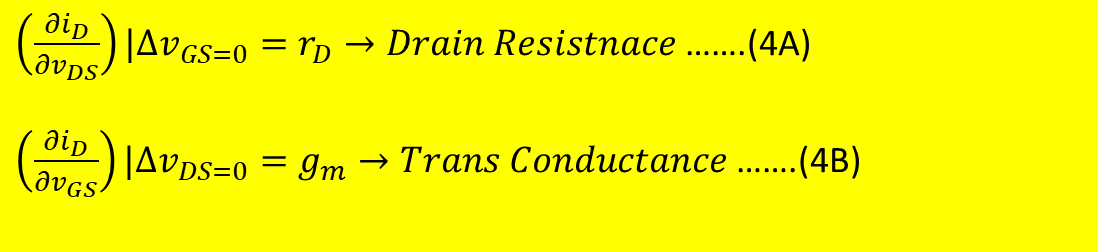### Definition of Parameters

Definition of Parameters:Substituting the equation (4) in equation (3) we get,Differentiate the equation (2), we getIn this equation,

∆VD=0, because VD is a constant

This leads us to the following equation (7):From this equation, the change in the variable value vDS is equal to the variation in the AC value vds.

Since vds is a variable value we will get the equation (8)